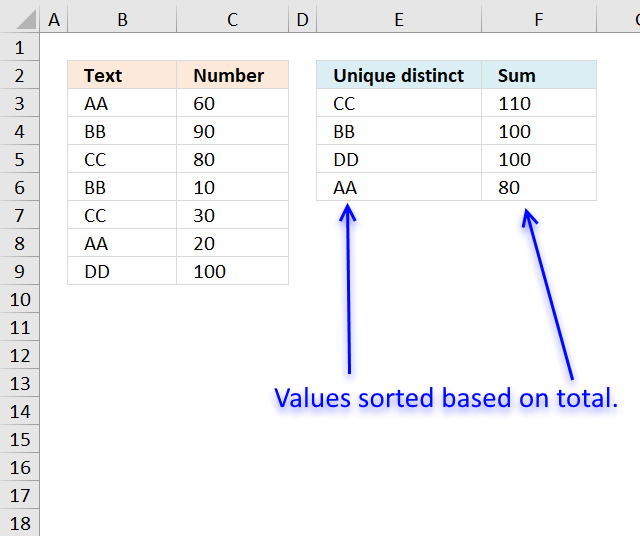Author: Oscar Cronquist Article last updated on December 06, 2018Filtering unique distinct text values and sort them based on the sum of adjacent values can easily be done by creating a pivot table, and I highly recommend it.

But in this blog post I want to show how to do this using an Excel array formula.

Array formula in E2:

=INDEX(\$A\$2:\$A\$8, MATCH(MAX(IF(NOT(COUNTIF(\$D\$1:D1, \$A\$2:\$A\$8)),SUMIF(\$A\$2:\$A\$8, "="&\$A\$2:\$A\$8, \$B\$2:\$B\$8),"")), IF(NOT(COUNTIF(\$D\$1:D1, \$A\$2:\$A\$8)),SUMIF(\$A\$2:\$A\$8, "="&\$A\$2:\$A\$8, \$B\$2:\$B\$8),""), 0))

How to create an array formula

1. Copy above array formula
2. Double press with left mouse button on cell E3
3. Paste array formula
4. Press and hold Ctrl + Shift simultaneouslöy
5. Press Enter

Formula in cell F3:

=SUMIF(\$A\$2:\$A\$8,E3,\$B\$2:\$B\$8)

Explaining formula in cell E2

Step 1 - Count prior values above current cell

The COUNTIF function counts values based on a condition or criteria, if number is 0 (zero) then the corresponding value has not yet been displayed.

NOT(COUNTIF(\$D\$1:D1, \$A\$2:\$A\$8))

becomes

NOT(COUNTIF("Unique distinct", {"AA";"BB";"CC";"BB";"CC";"AA";"DD"}))

becomes

NOT({0;0;0;0;0;0;0})

The NOT function returns the boolean opposite to the given argument. The array contains no boolean values, however it does contain their numerical equivavents. TRUE = 1 and FALSE = 0 (zero).

NOT({0;0;0;0;0;0;0})

and returns

{TRUE; TRUE; TRUE; TRUE; TRUE; TRUE; TRUE}.

Step 2 - IF TRUE then return the corresponding sum

The IF function returns the total if boolean value is TRUE. FALSE returns "" (nothing).

IF(NOT(COUNTIF(\$E\$2:E2, \$B\$3:\$B\$9)),SUMIF(\$B\$3:\$B\$9, "="&\$B\$3:\$B\$9, \$C\$3:\$C\$9),"")

becomes

IF({TRUE; TRUE; TRUE; TRUE; TRUE; TRUE; TRUE}, SUMIF(\$B\$3:\$B\$9, "="&\$B\$3:\$B\$9, \$C\$3:\$C\$9),"")

The SUMIF function adds numbers and returns a total based on a condition or criteria.

IF({TRUE; TRUE; TRUE; TRUE; TRUE; TRUE; TRUE}, SUMIF(\$B\$3:\$B\$9, "="&\$B\$3:\$B\$9, \$C\$3:\$C\$9),"")

becomes

IF({TRUE; TRUE; TRUE; TRUE; TRUE; TRUE; TRUE}, {80;100;110;100;110;80;100},"")

and returns

{80;100;110;100;110;80;100}.

Step 3 - Get the largest number in array

The MAX function returns the largest number in array ignoring blanks and text values.

MAX(IF(NOT(COUNTIF(\$E\$2:E2, \$B\$3:\$B\$9)),SUMIF(\$B\$3:\$B\$9, "="&\$B\$3:\$B\$9, \$C\$3:\$C\$9),""))

becomes

MAX({80;100;110;100;110;80;100})

and returns 110.

Step 4 - Match number

The MATCH function returns the relative position of a value in a cell range or array.

MATCH(MAX(IF(NOT(COUNTIF(\$E\$2:E2, \$B\$3:\$B\$9)),SUMIF(\$B\$3:\$B\$9, "="&\$B\$3:\$B\$9, \$C\$3:\$C\$9),"")), IF(NOT(COUNTIF(\$E\$2:E2, \$B\$3:\$B\$9)),SUMIF(\$B\$3:\$B\$9, "="&\$B\$3:\$B\$9, \$C\$3:\$C\$9),""), 0)

becomes

MATCH(110, IF(NOT(COUNTIF(\$E\$2:E2, \$B\$3:\$B\$9)),SUMIF(\$B\$3:\$B\$9, "="&\$B\$3:\$B\$9, \$C\$3:\$C\$9),""), 0)

becomes

MATCH(110, {80;100;110;100;110;80;100}, 0)

and returns 3.

Step 5 - Get value

The INDEX function returns a value based on row number (and column number if needed)

INDEX(\$A\$2:\$A\$8, MATCH(MAX(IF(NOT(COUNTIF(\$D\$1:D1, \$A\$2:\$A\$8)),SUMIF(\$A\$2:\$A\$8, "="&\$A\$2:\$A\$8, \$B\$2:\$B\$8),"")), IF(NOT(COUNTIF(\$D\$1:D1, \$A\$2:\$A\$8)),SUMIF(\$A\$2:\$A\$8, "="&\$A\$2:\$A\$8, \$B\$2:\$B\$8),""), 0))

becomes

INDEX(\$A\$2:\$A\$8, 3)

and returns "CC" in cell E2.

Get Excel *.xlsx file

Filter unique distinct list sorted based on sum of adjacent values.xlsx

Filtering unique distinct text values and sort them based on sum of adjacent values using a criteria listArray formula in cell E7:

=INDEX(\$A\$2:\$A\$8, MATCH(MAX(IF(NOT(COUNTIF(\$D\$6:D6, \$A\$2:\$A\$8)+(COUNTIF(\$E\$1:\$E\$2,\$A\$2:\$A\$8)=0)),SUMIF(\$A\$2:\$A\$8, "="&\$A\$2:\$A\$8, \$B\$2:\$B\$8),"")), IF(NOT(COUNTIF(\$D\$6:D6, \$A\$2:\$A\$8)+(COUNTIF(\$E\$1:\$E\$2,\$A\$2:\$A\$8)=0)),SUMIF(\$A\$2:\$A\$8, "="&\$A\$2:\$A\$8, \$B\$2:\$B\$8),""), 0))

Formula in cell E7:

=SUMIF(\$A\$2:\$A\$8,D7,\$B\$2:\$B\$8)